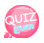# Creating Function

We discussed how to use different kinds of functions.
You can also create your own functions.
As we used before, frequently used functions can be made into functions and called whenever needed. Then we'll learn how to make a function.

## How to create a function

```function function name() {
}```

Now is it simple?
So let's create a function that tells us the sum of two numbers.
Let's call the function plus.

```function plus(\$num, \$num2) {
echo \$num + \$num2;
}```

In the code above, I created a plus function, and there are num and num2 variables in parentheses.
These variables are called parameters. In Korean, it's called a parameter.
So let's run the code

```<?php
function plus(\$num, \$num2) {
echo \$num + \$num2;
}
?>```

Running the code above does not produce any results. The reason is, of course, because we just declared it and didn't call it.
You already know how to call a function.

## How to call a function

`function name();`

The following is an example of including the code that calls the plus function.

```<?php
function plus(\$num, \$num2) {
echo \$num + \$num2;
}

plus(100,40);
?>```

Here is the result:Note that the declaration position of a function is not very relevant to the function.
Calling force first and declaring a function afterwards will not cause problems in execution.
Like this:

```<?php
plus(100,40);

function plus(\$num, \$num2) {
echo \$num + \$num2;
}
?>```

If you look at the plus function, there is an echo statement inside the function.
The value calculated in the function should be returned as the part of the function call.
Next, we'll learn how to return the value calculated by the function.